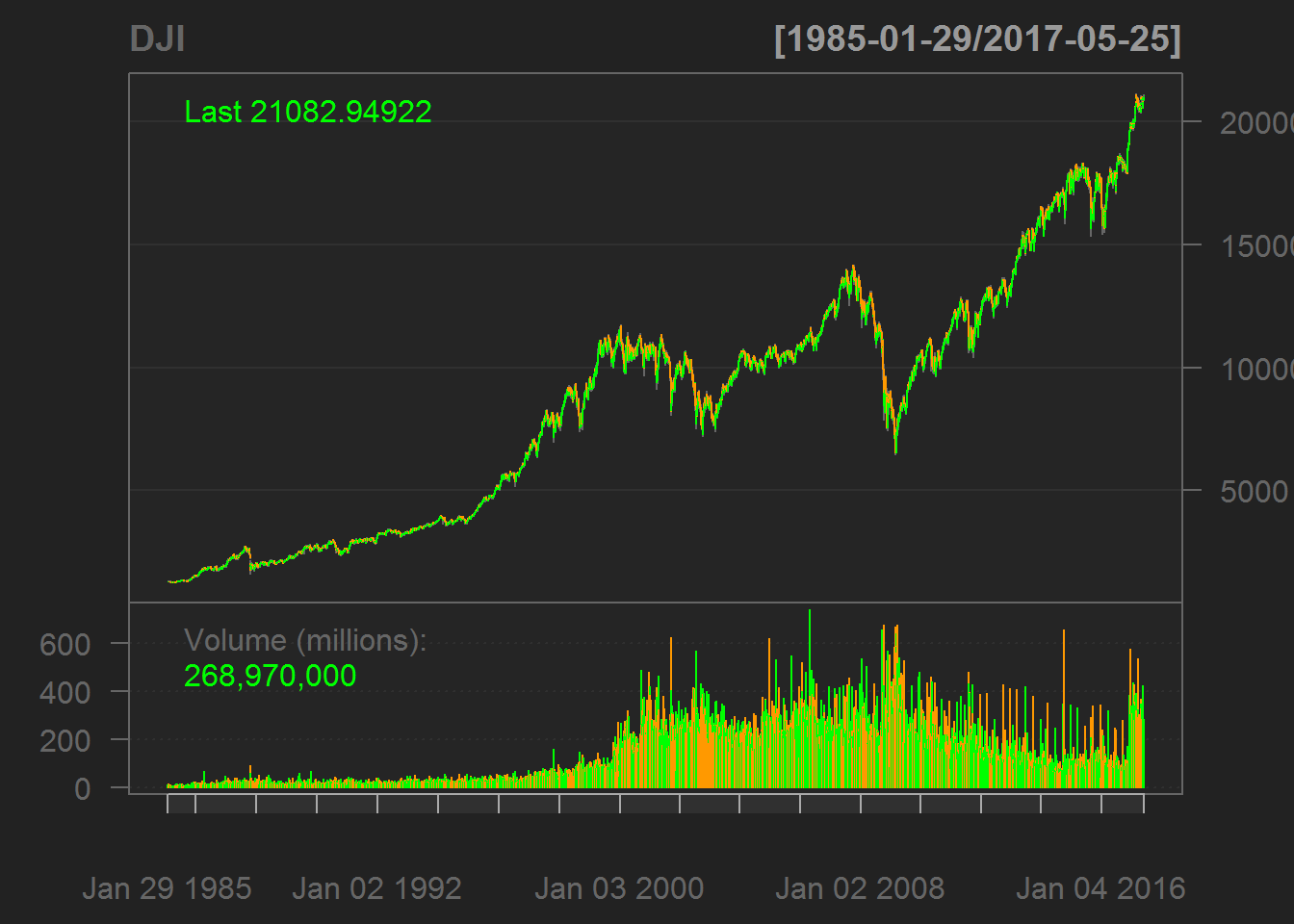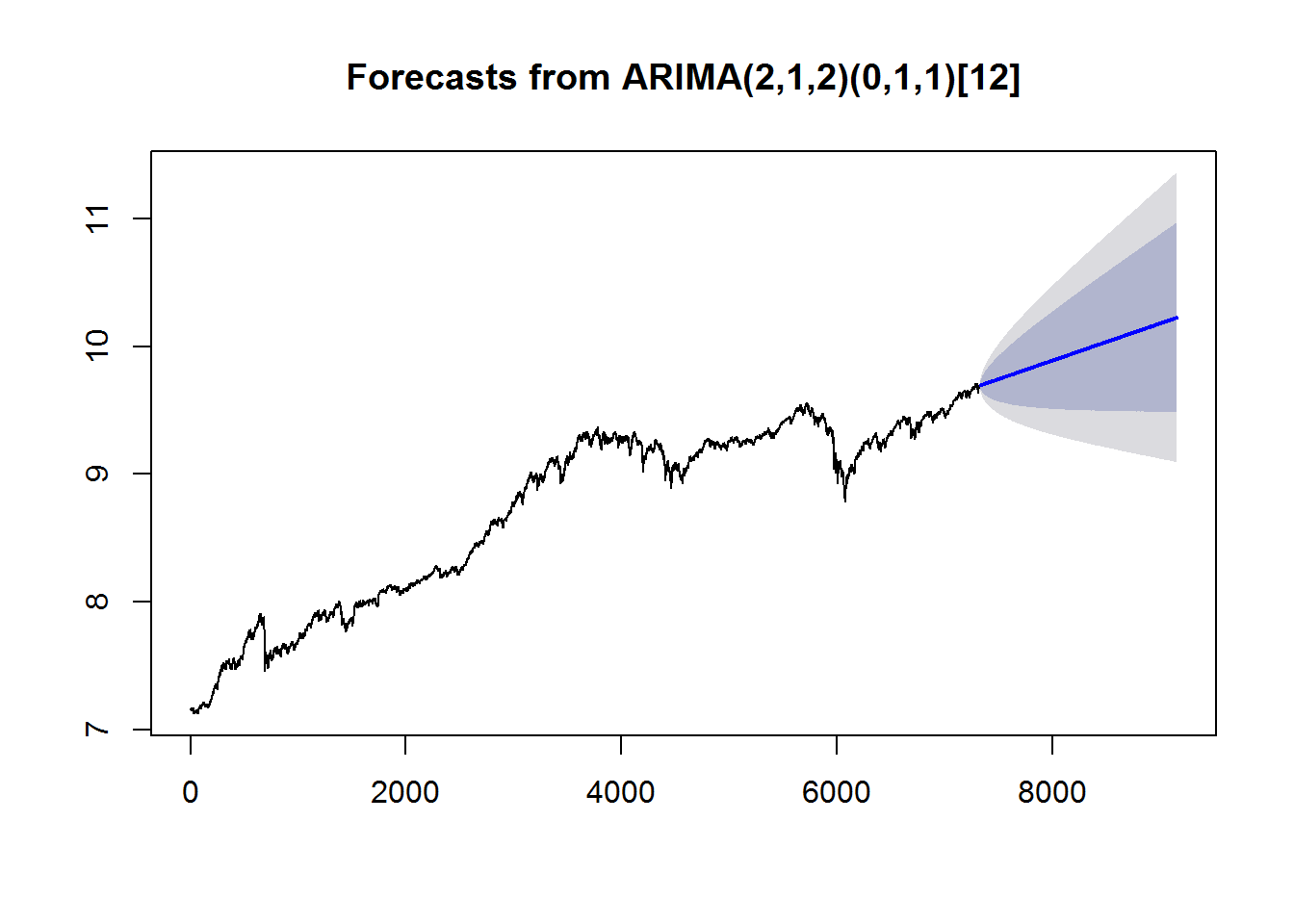### TIME SERIES - FINANCIAL DATA:

From this R-bloggers.

library(quantmod)
library(tseries)
library(forecast)

DJI$Date <- as.Date(DJI$Date,format="%m/%d/%Y")
rownames(DJI) <- DJI$Date DJI$Date <- NULL
head(DJI)
##               Open    High     Low   Close Adj.Close   Volume
## 1985-01-29 1277.72 1295.49 1266.89 1292.62   1292.62 13560000
## 1985-01-30 1297.37 1305.10 1278.93 1287.88   1287.88 16820000
## 1985-01-31 1283.24 1293.40 1272.64 1286.77   1286.77 14070000
## 1985-02-01 1276.94 1286.11 1269.77 1277.72   1277.72 10980000
## 1985-02-04 1272.08 1294.94 1268.99 1290.08   1290.08 11630000
## 1985-02-05 1294.06 1301.13 1278.60 1285.23   1285.23 13800000
d = DJI$Adj.Close summary(d) ## Min. 1st Qu. Median Mean 3rd Qu. Max. ## 1242 3398 9334 8613 11520 21120 chartSeries(DJI)adf.test(d)  ## ## Augmented Dickey-Fuller Test ## ## data: d ## Dickey-Fuller = -1.8305, Lag order = 20, p-value = 0.6502 ## alternative hypothesis: stationary The dataset is not stationary. We will difference and log: dow = 100 * diff(log(d))[-1] summary(dow) ## Min. 1st Qu. Median Mean 3rd Qu. Max. ## -25.63000 -0.44700 0.05353 0.03431 0.56240 10.51000 adf.test(dow) ## ## Augmented Dickey-Fuller Test ## ## data: dow ## Dickey-Fuller = -20.777, Lag order = 20, p-value = 0.01 ## alternative hypothesis: stationary Now it is stationary. We can fit a model using the Yule-Walker equations summary(arma(dow, order = c(2,2))) ## ## Call: ## arma(x = dow, order = c(2, 2)) ## ## Model: ## ARMA(2,2) ## ## Residuals: ## Min 1Q Median 3Q Max ## -25.97387 -0.47220 0.02879 0.53071 9.93999 ## ## Coefficient(s): ## Estimate Std. Error t value Pr(>|t|) ## ar1 -0.12861 0.19944 -0.645 0.5190 ## ar2 0.12745 0.18311 0.696 0.4864 ## ma1 0.09203 0.19737 0.466 0.6410 ## ma2 -0.18879 0.17934 -1.053 0.2925 ## intercept 0.03440 0.01592 2.161 0.0307 * ## --- ## Signif. codes: 0 '***' 0.001 '**' 0.01 '*' 0.05 '.' 0.1 ' ' 1 ## ## Fit: ## sigma^2 estimated as 1.228, Conditional Sum-of-Squares = 9997.75, AIC = 24800.96 So both the AR and MA coefficients are significant. We can divide instead the data into training and testing sets ($$90\,%$$ v $$10\%$$), fit the model in the training data, and then assess the performance on the testing set: train = d[1 : (0.9 * length(dow))] test = d[(0.9 * length(dow) + 1): length(dow)] fit = arima(log(train), c(2, 1, 2), seasonal=list(order = c(0, 1, 1), period=12)) predi = predict(fit, n.ahead = (length(dow) - (0.9*length(dow))))$pred
fore  = forecast(fit, h = 365 * 5) # Five years ahead

plot(fore)Well, it is an illustration. However, the accuracy assessment is not great:

accuracy(predi, test)
##                ME    RMSE      MAE      MPE     MAPE
## Test set 17855.26 17899.3 17855.26 99.94482 99.94482

If we calculate without using the i=1 middle value in arima:

dow = 100 * diff(log(d))[-1] # The difference taken outside the arima() call. 100 probably aides in computing since the numbers are very close to zero.
train = dow[1 : (0.9 * length(dow))]
test  = dow[(0.9 * length(dow) + 1):  length(dow)]

fit = arima(train, order = c(2, 0, 2), seasonal=list(order = c(0, 1, 1), period=12))

predi = predict(fit, n.ahead = (length(dow) - (0.9*length(dow))))\$pred
accuracy(predi, test)
##                   ME      RMSE       MAE MPE MAPE
## Test set 0.003215416 0.7946501 0.5670157 NaN  Inf

At this point I’m not sure how to evaluate these differences in output between both apparently equivalent systems.